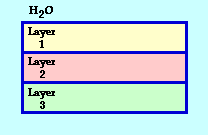Ray Optics: Refraction and Lenses

Refraction and Lenses: Audio Guided Solution

Problem 10:The diagram at the right shows series of transparent materials that form layers on top of each other and are surrounded by water (n=1.33). Layer 1 has an index of refraction of 1.91; layer 2 has an index of refraction of 1.52; layer 3 has an index of refraction of 1.36. A light ray in water approaches the boundary with layer 1 at 62.8 degrees.
a. Determine the angles of refraction for the light as it enters into each layer
b. Determine the angle of incidence of the light after it passes through each layer and strikes the boundary with the next layer.
c. Determine the angle of refraction for the light as it refracts out of layer 3 into the water.

Audio Guided Solution

Click to show or hide the answer!

Habits of an Effective Problem Solver

• Read the problem carefully and develop a mental picture of the physical situation. If necessary, sketch a simple diagram of the physical situation to help you visualize it.
• Identify the known and unknown quantities and record them in an organized manner. Equate given values to the symbols used to represent the corresponding quantity - e.g., do = 24,8 cm; di = 16.7 cm; f = ???.
• Use physics formulas and conceptual reasoning to plot a strategy for solving for the unknown quantity.
• Identify the appropriate formula(s) to use.
• Perform substitutions and algebraic manipulations in order to solve for the unknown quantity.